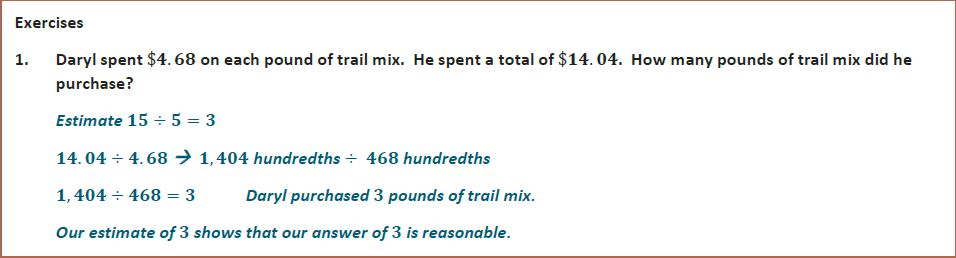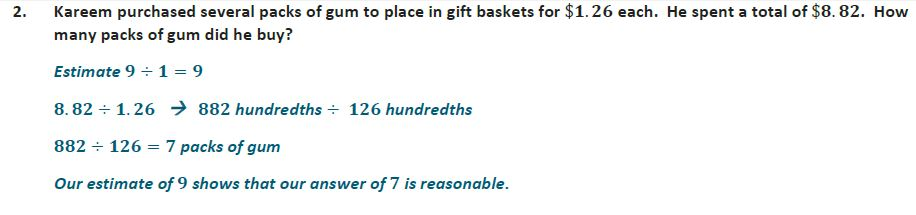6th Module 2--Arithmetic Operations Including Dividing by a Fraction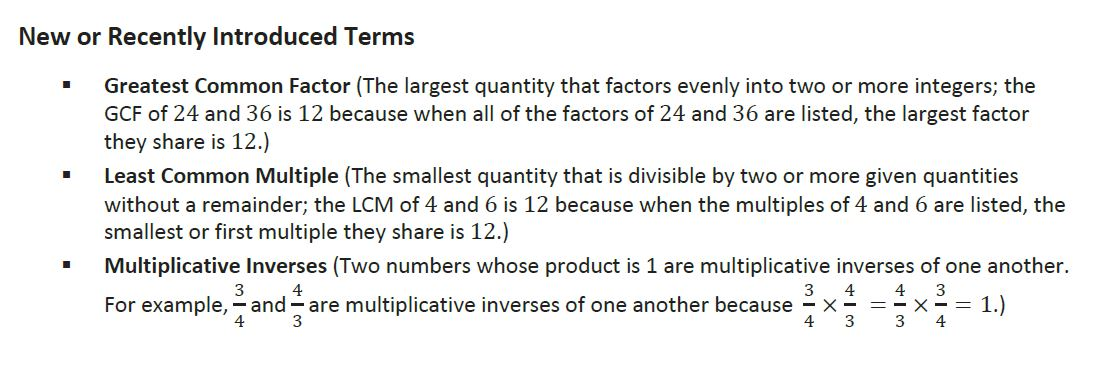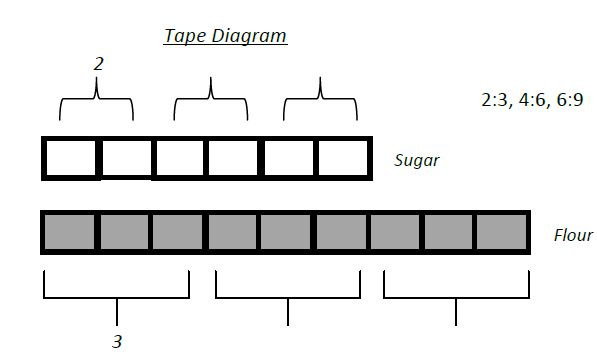Topic A: Divide a Fraction by Fraction

Divide a Fraction by Fraction Video

Divide a Whole Number by a Fraction Video

Divide a Fraction by a Whole Number

Interactive Fraction Tiles

IXL Practice:
1. W.1 Divide by fractions - with models
2. W.2 Reciprocals
3. W.3 Divide fractions
4. W.4 Estimate quotients when dividing mixed numbers
5. W.5 Divide fractions and mixed numbers
6. W.6 Divide fractions and mixed numbers: word problems

Topic B: Multi-Digit Decimal Operations--Adding, Subtracting, and Multiplying

Lesson 9: Sums and Differences of Decimals

1.Add Multi-Digit Decimals Using the Standard Algorithm Video

Subtract Multi-Digit Decimals Using the Standard Algorithm Video

Lesson 10: The Distributive Property and the Product of Decimals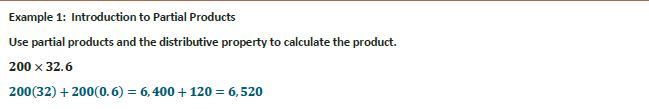Lesson 11: Fraction Multiplication and the Products of Decimals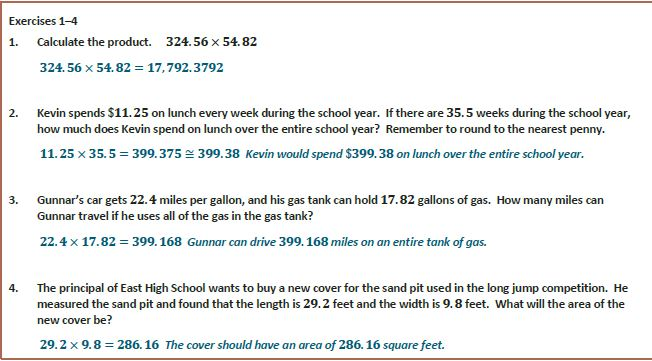Multiply Multi-Digit Decimals Using the Standard Algorithm Video

IXL:
Topic C: Dividing Whole Numbers and Decimals

Euclid's Division Algorithm

Lesson 12: Estimating Digits in a QuotientLesson 13: Dividing Multi-Digit Numbers Using the Algorithm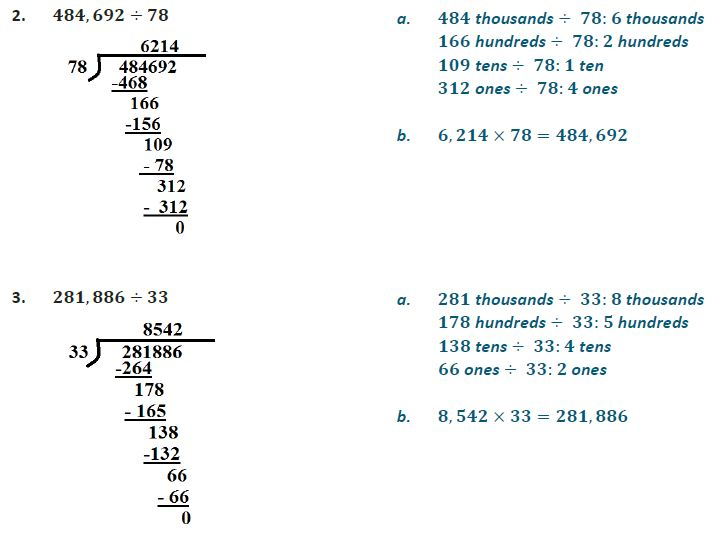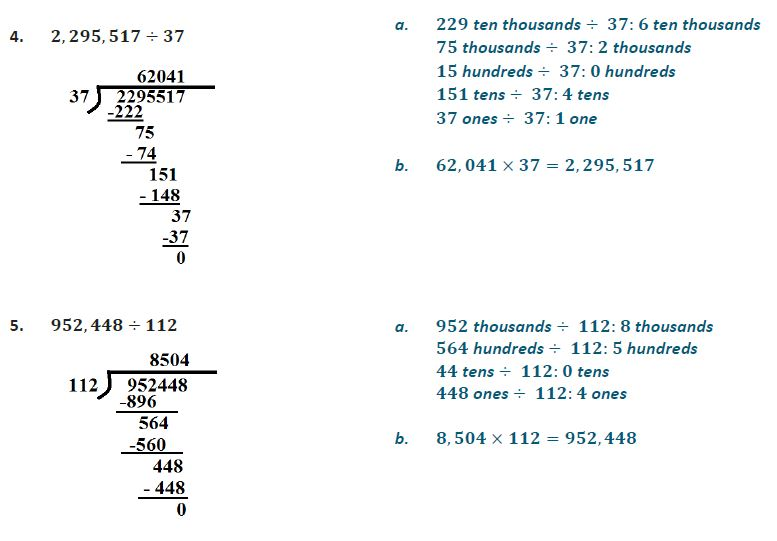Divide Multi-Digit Decimals Using the Standard Algorithm Video

IXL Practice:

Lesson 14: The Division Algorithm--Converting Decimal Division into Whole Number Division Using Fractions